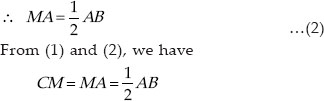Quadrilaterals (Mathematics) Class 9 - NCERT Questions
Q 1.

The angles of a quadrilateral are in the ratio 3:5:9:13. Find all the angles of a quadrilateral.

SOLUTION:

Let the angles of a quadrilateral be 3x, 5x, 9x and 13x.
∴ 3x + 5x + 9x + 13x = 360°
[Angle sum property of a quadrilateral]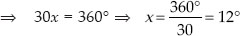∴ 3x = 3 × 12° = 36°
5x = 5 × 12° = 60°
9x = 9 × 12° = 108°
13x = 13 × 12° = 156°
∴ The required angles of a quadrilateral are 36°, 60°, 108° and 156°.

Q 2.

If the diagonals of a parallelogram are equal, then show that it is a rectangle.

SOLUTION:

ABCD is a parallelogram such that AC = BD.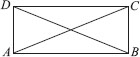In ∆ABC and ∆DCB,
AC = DB [Given] AB = DC
[Opposite sides of a parallelogram]
BC = CB [Common]
∴ ∆ABC ≅ ∆DCB [By SSS congruency]
⇒ ∥ABC = ∥DCB [By C.P.C.T.] …(1)
Now, AB ∥ DC and BC is a transversal.
[∵ ABCD is a parallelogram]
∴ ∠ABC + ∠DCB = 180° …(2)
[Co-interior angles]
From (1) and (2), we have ∠ABC = ∠DCB = 90°
i.e. ABCD is a parallelogram having an angle equal to 90°.
∴ ABCD is a rectangle.

Q 3.

Show that if the diagonals of a quadrilateral bisect each other at right angles, then it is a rhombus.

SOLUTION:

We have a quadrilateral ABCD such that the diagonals AC and BD bisect each other at right angles at O.
∴ In ∆AOB and ∆AOD, we have
AO = OA [Common]
OB = OD [O is the midpoint of BD]
∠AOB = ∠AOD [Each 90°]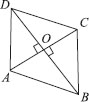∴ ∆AOB ≅ ∆AOD [By SAS congruency]
∴ AB = AD [By C.P.C.T.] …(1)
Similarly, AB = BC …(2)
BC = CD …(3)
CD = DA …(4)
∴ From (1), (2), (3) and (4), we have
AB = BC = CD = DA
Thus, a quadrilateral ABCD is a rhombus.
Alternatively : ABCD can be proved first a parallelogram then proving one pair of adjacent sides equal will results in rhombus

Q 4.

Show that the diagonals of a square are equal and bisect each other at right angles.

SOLUTION:

We have a square ABCD such that its diagonals AC and BD intersect at O.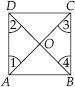(i) To prove that the diagonals are equal, i.e., AC = BD. In ∆ABC and ∆BAD, we have
AB = BA [Common]
BC = AD [Sides of a square ABCD]
∠ABC = ∠BAD [∵ each angle is 90°]
∴ ∆ABC ≅ ∆BAD [By SAS congruency]
⇒ AC = BD [By C.P.C.T.] ...(1)
(ii) ∵ AD ∥ BC and AC is a transversal.
[∵ A square is a parallelogram]
∴ ∠1 = ∠3
[Alternate interior angles are equal]
Similarly, ∠2 = ∠4
Now, in ∆OAD and ∆OCB, we have
AD = CB [Sides of a square ABCD]
∠1 = ∠3 [Proved]
∠2 = ∠4 [Proved]
∴ ∆OAD ≅ ∆OCB [By ASA congruency]
⇒ OA = OC and OD = OB [By C.P.C.T.]
i.e., the diagonals AC and BD bisect each other at O. …(2)
(iii) In ∆OBA and ∆ODA, we have
OB = OD [Proved]
BA = DA [Sides of a square]
OA = AO [Common]
∴ ∆OBA ≅ ∆ODA [By SSS congruency]
⇒ ∠AOB = ∠AOD …(3)
[By C.P.C.T.]
∵ ∠AOB and ∠AOD form a linear pair.
∴ ∠AOB + ∠AOD = 180°
∴ ∠AOB = ∠AOD = 90° [By(3)]
⇒ AC ⊥ BD …(4)
From (1), (2) and (4), we get AC and BD are equal and bisect each other at right angles.

Q 5.

Show that if the diagonals of a quadrilateral are equal and bisect each other at right angles, then it is a square.

SOLUTION:

We have a quadrilateral ABCD such that O is the midpoint of AC and BD.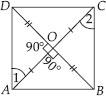Also, AC ⊥ BD.
Now, in ∆AOD and ∆AOB, we have
∠AOD = ∠AOB [Each 90°]
AO = OA [Common]
OD = OB [∵ O is the midpoint of BD]
∴ ∆AOD ≅ ∆AOB [By SAS congruency]
⇒ AD = AB [By C.P.C.T.] …(1)
Similarly, we have AB = BC …(2)
BC = CD …(3)
CD = DA …(4)
From (1), (2), (3) and (4), we have
AB = BC = CD = DA
∴ Quadrilateral ABCD have all sides equal.
In ∆AOD and ∆COB, we have
AO = CO [Given]
OD = OB [Given]
∠AOD = ∠COB
[Vertically opposite angles]
So, ∆AOD ≅ ∆COB [By SAS congruency]
∴ ∠1 = ∠2 [By C.P.C.T.]
But, they form a pair of alternate interior angles.
Similarly, AB ∥ DC
∴ ABCD is a parallelogram
∴ Parallelogram having all its sides equal is a rhombus.
∴ ABCD is a rhombus.
Now, in ∆ABC and ∆BAD, we have
AC = BD [Given]
AB = BA [Common]
∴ ∆ABC ≅ ∆BAD [By SSS congruency]
∴ ∠ABC = ∠BAD [By C.P.C.T.] …(5)
Since, AD ∥ BC and AB is a transversal.
∴ ∠ABC + ∠BAD = 180° …(6)
⇒ ∠ABC = ∠BAD = 90° [By (5) & (6)]
So, rhombus ABCD is having one angle equal to 90°.
Thus, ABCD is a square.

Q 6.

Diagonal AC of a parallelogram ABCD bisects ∠A(see figure). Show that
(i) it bisects ∠C also.
(ii)ABCD is a rhombus.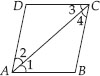SOLUTION:

We have a parallelogram ABCD in which diagonal AC bisects ∠A ⇒ ∠DAC = ∠BAC
(i) Since, ABCD is a parallelogram.
∴ AB ∥ DC and AC is a transversal.
∴ ∠1 = ∠3 [∵ alternate interior angles are equal] …(1)
Also, BC ∥ AD and AC is a transversal.
∴ ∠2 = ∠4 [Alternate interior angles] …(2)
Also, ∠1 = ∠2 [∵ AC bisects ∠A] …(3)
From (1), (2) and (3), we have
∠3 = ∠4 ⇒ AC bisects ∠C.
(ii) In ΔABC, we have
∠1 = ∠4 [From (2) and (3)]
⇒ BC = AB …(4)
[∵ sides opposite to equal angles of a ∆ are equal]
But, ABCD is a parallelogram [Given]
∴ AB = DC …(6)
From (4), (5) and (6), we have
AB = BC = CD = DA
Thus, ABCD is a rhombus.

Q 7.

ABCD is a rhombus. Show that diagonal AC bisects ∠A as well as ∠C and diagonal BD bisects ∠B as well as ∠D.

SOLUTION:

Since ABCD is a rhombus
⇒ AB = BC = CD = DA
Also, AB ∥ CD and AD ∥ BC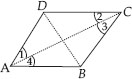Now, AD = CD ⇒ ∠1 = ∠2 …(1)
[∵ angles opposite to equal sides of a triangle are equal]
Also, AD BC and AC is the transversal.
[∵ every rhombus is a parallelogram]
∠1 = ∠3 …(2)
[∵ Alternate interior angles are equal]
From (1) and (2), we have
∠2 = ∠3 …(3)
Since, AB DC and AC is transversal.
∴ ∠2 = ∠4 …(4)
[∵ Alternate interior angles are equal]
From (1) and (4), we have
∠1 = ∠4
⇒ AC bisects ∠C as well as ∠A.
Similarly, we can prove that BD bisects ∠B as well as ∠D.

Q 8.

ABCD is a rectangle in which diagonal AC bisects ∠A as well as ∠C. Show that:
(i) ABCD is a square.
(ii) diagonal BD bisects ∠B as well as ∠D.

SOLUTION:

We have a rectangle ABCD such that AC bisects ∠A as well as ∠C.
i.e. ∠1 = ∠4 and ∠2 = ∠3 …(1)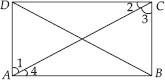(i) Since, every rectangle is a parallelogram.
∴ ABCD is a parallelogram.
⇒ AB ∥ CD and AC is a transversal.
∴ ∠2 = ∠4 [Alternate interior angles] …(2)
From (1) and (2), we have
∠3 = ∠4
In ΔABC, ∠3 = ∠4
⇒ AB = BC
[∵ sides opposite to equal angles of a ∆ are equal]
⇒ ABCD is a rectangle having adjacent sides equal.
⇒ ABCD is a square.
(ii) Since, ABCD is a square and diagonals of a square bisect the opposite angles.
So, BD bisects ∠B as well as ∠D.

Q 9.

In a parallelogram ABCD, two points P and Q are taken on diagonal BD such that DP = BQ (see figure). Show that:
(i) ∆APD ≅ ∆CQB
(ii) AP = CQ
(iii) ∆AQB ≅ ∆CPD (iv) AQ = CP
(v) APCQ is a parallelogram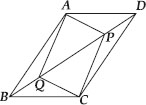SOLUTION:

We have parallelogram ABCD, BD is the diagonal and points P and Q are such that
PD = QB [Given]
(i) Since, AD ∥ BC and BD is a transversal.
[∵ Alternate interior angles are equal]
Now, in ∆APD and ∆CQB, we have
AD = CB [Opposite sides of a parallelogram
ABCD are equal]
PD = QB [Given]
∴ ∆APD ≅ ∆CQB [By SAS congruency]
(ii) Since, ∆APD ≅ ∆CQB [Proved]
⇒ AP = CQ [By C.P.C.T.]
(iii) Since, AB ∥ CD and BD is a transversal.
∴ ∠ABD = ∠CDB
⇒ ∠ABQ = ∠CDP
Now, in ∆AQB and ∆CPD, we have
QB = PD [Given]
∠ABQ = ∠CDP [Proved]
AB = CD [∵ Opposite sides of a parallelogram ABCD are equal]
∴ ∆AQB ≅ ∆CPD [By SAS congruency]
(iv) Since, ∆AQB ≅ ∆CPD [Proved]
⇒ AQ = CP [By C.P.C.T.]
(v) ∵ In a quadrilateral APCQ
Opposite sides are equal. [Proved]
∴ APCQ is a parallelogram.

Q 10.

ABCD is a parallelogram and AP and CQ are perpendiculars from vertices A and C on diagonal BD (see figure). Show that :
(i) ∆APB≅ ∆CQD
(ii) AP = CQ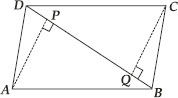SOLUTION:

(i) In ∆APB and ∆CQD, we have
∠APB = ∠CQD [Each 90°]
AB = CD [∵ Opposite sides of a parallelogram ABCD are equal]
∠ABP = ∠CDQ [∵ Alternate angles are euqal as AB∥ CD and BD is a transversal]
∴ ∆APB ≅ ∆CQD [By AAS congruency]
(ii) Since, ∆APB ≅ ∆CQD [Proved]
⇒ AP = CQ [By C.P.C.T.]

Q 11.

In ∆ABC and ∆DEF, AB = DE, AB ∥DE, BC = EF and BC ∥ EF. Vertices A, B and C are joined to vertices D, E and F respectively (see figure). Show that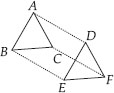(i) quadrilateral ABED is a parallelogram.
(ii) quadrilateral BEFC is a parallelogram.
(iv) quadrilateral ACFD is a parallelogram.
(v) AC = DF

SOLUTION:

(i) We have
AB = DE [Given]
AB DE [Given]
i.e., ABED is a quadrilateral in which a pair of opposite sides (AB and DE) are parallel and of equal length.
∴ ABED is a parallelogram.
(ii) BC = EF [Given]
and BC ∥ EF [Given]
i.e. BEFC is a quadrilateral in which a pair of opposite sides (BC and EF) are parallel and of equal length.
∴ BEFC is a parallelogram.
(iii) ABED is a parallelogram [Proved]
∴ AD ∥ BE and AD = BE [∵ Opposite sides of a parallelogram are equal and parallel] …(1)
Also, BEFC is a parallelogram. [Proved]
∴ BE ∥ CF and BE = CF [∵ Opposite sides of a parallelogram are equal and parallel] …(2)
From (1) and (2), we have
i.e., In quadrilateral ACFD, one pair of opposite sides (AD and CF) are parallel and equal in length.
∴ Quadrilateral ACFD is a parallelogram.
(v) Since, ACFD is a parallelogram.
[Proved]
So, AC = DF [∵ opposite sides of a parallelogram are equal]
(vi) In ∆ABC and ∆DEF, we have
AB = DE [∵ opposite sides of a parallelogram ABED are equal]
BC = EF [∵ opposite sides of a parallelogram BEFC are equal]
AC = DF [Proved in (v)]
∴ ∆ABC ≅ ∆DEF [By SSS congruency]

Q 12.

ABCD is a trapezium in which AB ∥ CD and AD = BC (see figure). Show that
(i) ∠A = ∠ B
(ii) ∠C = ∠D
(iv) Diagonal AC = Diagonal BDHint: Extend AB and draw a line through C parallel to DA intersecting AB produced at E.

SOLUTION:

(i) Produce AB to E and draw CE ∥ AD.
∵ AB ∥ DC ⇒ AE DC
Also AD ∥ CE [By construction]
∴ AECD is a parallelogram.
⇒ AD = CE …(1) [∵opposite sides of the parallelogram AECD are equal]
But AD = BC …(2) [Given]
By (1) and (2)
BC = CE
Now, in ∆BCE, we have BC = CE
⇒ ∠CEB = ∠CBE ...(1)
[∵ angles opposite to equal sides of a triangle are equal]
Also, ∠ABC + ∠CBE = 180° …(2)
[Linear pair]
and ∠A + ∠CEB = 180° …(3)
[co-interior angles of a parallelogram ADCE
] From (2) and (3), we get
∠ABC + ∠CBE = ∠A + ∠CEB
⇒ ∠ABC = ∠A [From (1)]
⇒ ∠B = ∠A …(4)
(ii) AB ∥ CD and AD is a transversal.
∴ ∠A + ∠D = 180° …(5)
[Co-interior angles of a paralelogram]
Similarly, ∠B + ∠C = 180° …(6)
From (5) and (6), we get
∠A + ∠D = ∠B + ∠C
⇒ ∠C = ∠D [From (4)]
(iii) In ∆ABC and ∆BAD, we have
AB = BA [Common]
∴ ∆ABC ≅ ∆BAD [By SAS congruency]
(iv) Since, ∆ABC ≅ ∆BAD [Proved]
⇒ AC = BD [By C.P.C.T.]

Q 13.

ABCD is a quadrilateral in which P, Q, R and S are midpoints of the sides AB, BC, CD and DA (see figure). AC is a diagonal. Show that: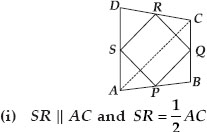(ii) PQ = SR
(iii) PQRS is a parallelogram.

SOLUTION:

(i) In ∆ACD, we have
S is the midpoint of AD and R is the midpoint of CD.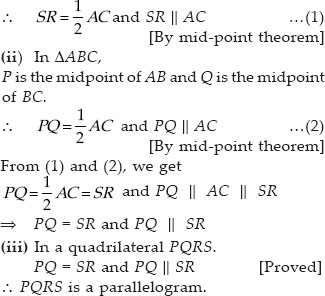Q 14.

ABCD is a rhombus and P, Q, R and S are the midpoints of the sides AB, BC, CD and DA respectively. Show that the quadrilateral PQRS is a rectangle.

SOLUTION:

Join AC. In ∆ABC, P and Q are the midpoints of AB and BC respectively.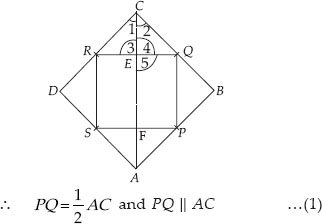[By mid-point theorem]
In ∆ADC, R and S are the midpoints of CD and DA respectively.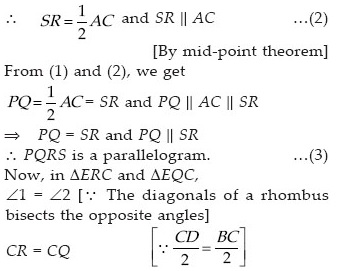CE = EC [Common]
∴ ∆ERC ≅ ∆EQC [By SAS congruency]
⇒ ∠3 = ∠4 …(4) [By C.P.C.T.]
But ∠3 + ∠4 = 180° …(5) [Linear pair]
From (4) and (5), we get
⇒ ∠3 = ∠4 = 90°
Now, ∠RQP = 180° – ∠5
[∵ Co-interior angles for PQ ∥ AC and EQ is transversal]
But ∠5 = ∠3 [∵ Vertically opposite angles are equal]
∴ ∠5 = 90°
So, ∠RQP = 180° – ∠5 = 90°
∴ One angle of parallelogram PQRS is 90°.
Thus, PQRS is a rectangle.

Q 15.

ABCD is a rectangle and P, Q, R and S are midpoints of the sides AB, BC, CD and DA respectively. Show that the quadrilateral PQRS is a rhombus.

SOLUTION: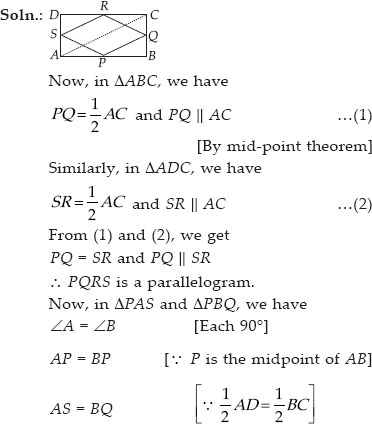∴ ∆PAS ≅ ∆PBQ [By SAS congruency]
⇒ PS = PQ [By C.P.C.T.]
Also, PS = QR and PQ = SR. [∵ opposite sides of parallelogram PQRS are equal]
So, PQ = QR = RS = SP
i.e., PQRS is a parallelogram having all of its sides equal.
Hence, PQRS is a rhombus.

Q 16.

ABCD is a trapezium in which AB ∥DC, BD is a diagonal and E is the midpoint of AD. A line is drawn through E parallel to AB intersecting BC at F (see figure). Show that F is the mid point
of BC.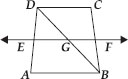SOLUTION:

In ∆DAB, We know that E is the midpoint of AD and EG ∥ AB [∵ EF ∥ AB]
∴ Using the converse of midpoint theorem, we get, G is the midpoint of BD.
Again in ∆BDC, we have
G is the midpoint of BD and GF ∥ DC.
[∵ AB ∥ DC and EF ∥ AB and GF is a part of EF]
Using the converse of the midpoint theorem, we get, F is the midpoint of BC.

Q 17.

In a parallelogram ABCD, E and F are the midpoints of sides AB and CD respectively (see figure). Show that the line segments AF and EC trisect the diagonal BD.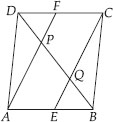SOLUTION:

Since, the opposite sides of a parallelogram are parallel and equal.
∴ AB ∥ DC ⇒ AE ∥ FC …(1)
and AB = DC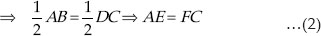From (1) and (2), we have
AE ∥ FC and AE = FC
∴ AECF is a parallelogram.
Now, in ∆ DQC, we have
F is the midpoint of DC and FP ∥ CQ
[∵ AF ∥ CE]
⇒ DP = PQ ...(3)
[By converse of mid-point theorem]
Similarly, in ∆ BAP, E is the mid-point of AB and EQ ∥ AP [∵ AF ∥ CE]
⇒ BQ = PQ …(4)
[By converse of mid-point theorem]
∴ From (3) and (4), we have
DP = PQ = BQ
So, the line segments AF and EC trisect the diagonal BD.

Q 18.

Show that the line segments joining the midpoints of the opposite sides of a quadrilateral bisect each other.

SOLUTION:

Join PQ, QR, RS and SP. Let us also join PR and SQ.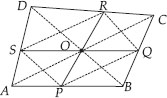Now, in ∆ABC, we have P and Q are the midpoints of its sides AB and BC respectively.
[By mid-point theorem]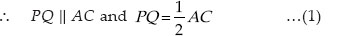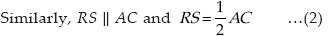∴ By (1) and (2), we get
PQ ∥ RS, PQ = RS
∴ PQRS is a parallelogram.
And the diagonals of a parallelogram bisect each other, i.e., PR and SQ bisect each other.
Thus, the line segments joining the mid-points of opposite sides of a quadrilateral ABCD bisect each other.

Q 19.

ABC is a triangle right angled at C. A line through the midpoint M of hypotenuse AB and parallel to BC intersects AC at D. Show that
(i) D is the midpoint of AC
(ii) MD ⊥AC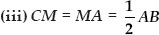SOLUTION:

(i) In ∆ACB, we have
M is the midpoint of AB. [Given]
MD ∥ BC [Given]
∴ Using the converse of mid-point theorem, D is the midpoint of AC.
(ii) Since, MD ∥ BC and AC is a transversal.
∴ ∠MDA = ∠BCA
[∵ Corresponding angles are equal]
As ∠BCA = 90° [Given]
∴ ∠MDA = 90°
⇒ MD ⊥ AC.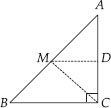(iii) In ∆ADM and ∆CDM, we have ∠ADM = ∠CDM [Each equal to 90°]
MD = DM [Common]
AD = CD [∵ D is the midpoint of AC]
∴ ∆ADM ≅ ∆CDM [By SAS congruency]
⇒ MA = MC [By C.P.C.T.] …(1)
∵ M is the midpoint of AB [Given]